# Selina solutions for Concise Mathematics Class 7 ICSE chapter 15 - Triangles [Latest edition]

#### Chapters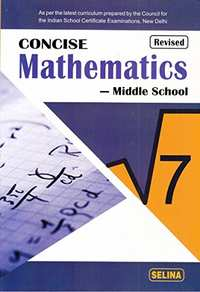## Chapter 15: Triangles

Exercise 15 (A)Exercise 15 (B)Exercise 15 (C)
Exercise 15 (A)

### Selina solutions for Concise Mathematics Class 7 ICSE Chapter 15 TrianglesExercise 15 (A)

Exercise 15 (A) | Q 1.1

State, if the triangle is possible with the following angles :

20°, 70°, and 90°

Exercise 15 (A) | Q 1.2

State, if the triangle is possible with the following angles :

40°, 130°, and 20°

Exercise 15 (A) | Q 1.3

State, if the triangle is possible with the following angles :

60°, 60°, and 50°

Exercise 15 (A) | Q 1.4

State, if the triangle is possible with the following angles :

125°, 40°, and 15°

Exercise 15 (A) | Q 2

If the angles of a triangle are equal, find its angles.

Exercise 15 (A) | Q 3

In a triangle ABC, ∠A = 45° and ∠B = 75°, find ∠C.

Exercise 15 (A) | Q 4

In a triangle PQR, ∠P = 60° and ∠Q = ∠R, find ∠R.

Exercise 15 (A) | Q 5.1

Calculate the unknown marked angles of the following figure :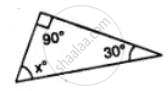Exercise 15 (A) | Q 5.2

Calculate the unknown marked angles of the following figure :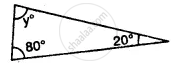Exercise 15 (A) | Q 5.3

Calculate the unknown marked angles of the following figure :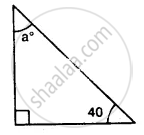Exercise 15 (A) | Q 6.1

Find the value of the angle in the given figure: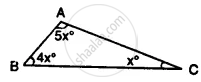Exercise 15 (A) | Q 6.2

Find the value of the angle in the given figure: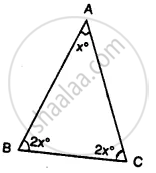Exercise 15 (A) | Q 7.1

Find the unknown marked angles in the given figure: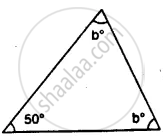Exercise 15 (A) | Q 7.2

Find the unknown marked angles in the given figure: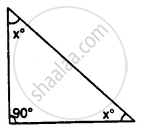Exercise 15 (A) | Q 7.3

Find the unknown marked angles in the given figure: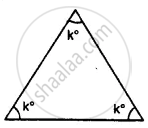Exercise 15 (A) | Q 7.4

Find the unknown marked angles in the given figure: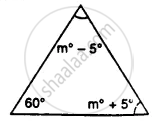Exercise 15 (A) | Q 8

In the given figure, show that: ∠a = ∠b + ∠c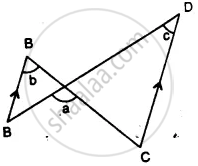(i) If ∠b = 60° and ∠c = 50° ; find ∠a.
(ii) If ∠a = 100° and ∠b = 55° : find ∠c.
(iii) If ∠a = 108° and ∠c = 48° ; find ∠b.

Exercise 15 (A) | Q 9

Calculate the angles of a triangle if they are in the ratio 4: 5: 6.

Exercise 15 (A) | Q 10

One angle of a triangle is 60°. The other two angles are in the ratio of 5: 7. Find the two angles.

Exercise 15 (A) | Q 11

One angle of a triangle is 61° and the other two angles are in the ratio 1 1/2: 1 1/3. Find these angles.

Exercise 15 (A) | Q 12.1

Find the unknown marked angles in the given figure: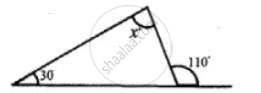Exercise 15 (A) | Q 12.2

Find the unknown marked angles in the given figure: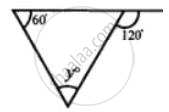Exercise 15 (A) | Q 12.3

Find the unknown marked angles in the given figure: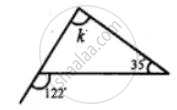Exercise 15 (A) | Q 12.4

Find the unknown marked angles in the given figure: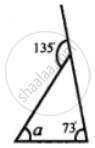Exercise 15 (A) | Q 12.5

Find the unknown marked angles in the given figure: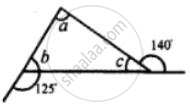Exercise 15 (A) | Q 12.6

Find the unknown marked angles in the given figure: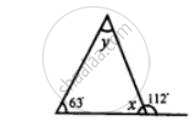Exercise 15 (A) | Q 12.7

Find the unknown marked angles in the given figure: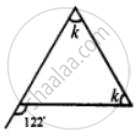Exercise 15 (A) | Q 12.8

Find the unknown marked angles in the given figure: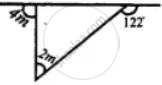Exercise 15 (A) | Q 12.9

Find the unknown marked angles in the given figure: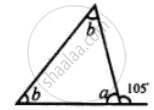Exercise 15 (B)

### Selina solutions for Concise Mathematics Class 7 ICSE Chapter 15 TrianglesExercise 15 (B)

Exercise 15 (B) | Q 1.1

Find the unknown angles in the given figure: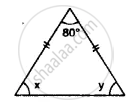Exercise 15 (B) | Q 1.2

Find the unknown angles in the given figure: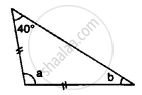Exercise 15 (B) | Q 1.3

Find the unknown angles in the given figure: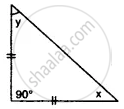Exercise 15 (B) | Q 1.4

Find the unknown angles in the given figure: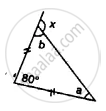Exercise 15 (B) | Q 1.5

Find the unknown angles in the given figure: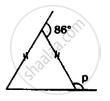Exercise 15 (B) | Q 1.6

Find the unknown angles in the given figure: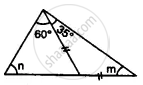Exercise 15 (B) | Q 1.7

Find the unknown angles in the given figure: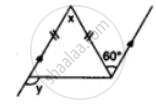Exercise 15 (B) | Q 2.1

Apply the properties of isosceles and equilateral triangles to find the unknown angles in the given figure: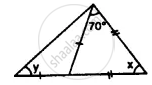Exercise 15 (B) | Q 2.2

Apply the properties of isosceles and equilateral triangles to find the unknown angles in the given figure: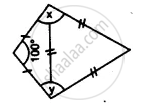Exercise 15 (B) | Q 2.3

Apply the properties of isosceles and equilateral triangles to find the unknown angles in the given figure: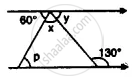Exercise 15 (B) | Q 2.4

Apply the properties of isosceles and equilateral triangles to find the unknown angles in the given figure: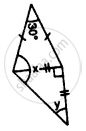Exercise 15 (B) | Q 2.5

Apply the properties of isosceles and equilateral triangles to find the unknown angles in the given figure: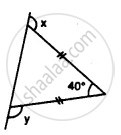Exercise 15 (B) | Q 2.6

Apply the properties of isosceles and equilateral triangles to find the unknown angles in the given figure: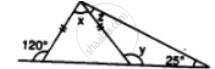Exercise 15 (B) | Q 3

The angle of a vertex of an isosceles triangle is 100°. Find its base angles.

Exercise 15 (B) | Q 4

One of the base angles of an isosceles triangle is 52°. Find its angle of the vertex.

Exercise 15 (B) | Q 5

In an isosceles triangle, each base angle is four times its vertical angle. Find all the angles of the triangle.

Exercise 15 (B) | Q 6

The vertical angle of an isosceles triangle is 15° more than each of its base angles. Find each angle of the triangle.

Exercise 15 (B) | Q 7

The base angle of an isosceles triangle is 15° more than its vertical angle. Find its each angle.

Exercise 15 (B) | Q 8

The vertical angle of an isosceles triangle is three times the sum of its base angles. Find each angle.

Exercise 15 (B) | Q 9

The ratio between a base angle and the vertical angle of an isosceles triangle is 1: 4. Find each angle of the triangle.

Exercise 15 (B) | Q 10

In the given figure, BI is the bisector of ∠ABC and Cl is the bisector of ∠ACB. Find ∠BIC.

Exercise 15 (B) | Q 11

In the given figure, express a in terms of b.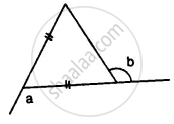Exercise 15 (B) | Q 12.1

In Figure, BP bisects ∠ABC and AB = AC. Find x.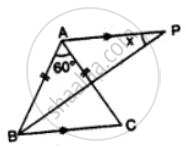Exercise 15 (B) | Q 12.2

Find x in Figure Given: DA = DB = DC, BD bisects ∠ABC and∠ADB = 70°.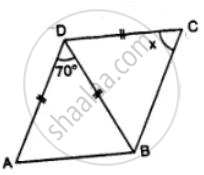Exercise 15 (B) | Q 13.1

In the figure, given below, ABCD is a square, and ∆ BEC is an equilateral triangle. Find, the case:∠ABE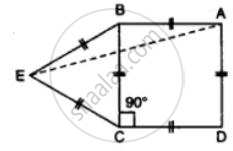Exercise 15 (B) | Q 13.2

In the figure, given below, ABCD is a square, and ∆ BEC is an equilateral triangle. Find, the case:∠BAE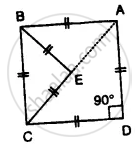Exercise 15 (B) | Q 14

In ∆ ABC, BA and BC are produced. Find the angles a and h. if AB = BC.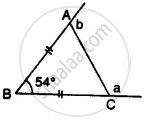Exercise 15 (C)

### Selina solutions for Concise Mathematics Class 7 ICSE Chapter 15 TrianglesExercise 15 (C)

Exercise 15 (C) | Q 1.1

Construct a ∆ABC such that:

AB = 6 cm, BC = 4 cm and CA = 5.5 cm

Exercise 15 (C) | Q 1.2

Construct a ∆ABC such that:

CB = 6.5 cm, CA = 4.2 cm and BA = 51 cm

Exercise 15 (C) | Q 1.3

Construct a ∆ABC such that:

BC = 4 cm, AC = 5 cm and AB = 3.5 cm

Exercise 15 (C) | Q 2.1

Construct a ∆ ABC such that:

AB = 7 cm, BC = 5 cm and ∠ABC = 60°

Exercise 15 (C) | Q 2.2

Construct a ∆ ABC such that:

BC = 6 cm, AC = 5.7 cm and ∠ACB = 75°

Exercise 15 (C) | Q 2.3

Construct a ∆ ABC such that:

AB = 6.5 cm, AC = 5.8 cm and ∠A = 45°

Exercise 15 (C) | Q 3.1

Construct a ∆ PQR such that :

PQ = 6 cm, ∠Q = 60° and ∠P = 45°. Measure ∠R.

Exercise 15 (C) | Q 3.2

Construct a ∆ PQR such that:

QR = 4.4 cm, ∠R = 30° and ∠Q = 75°. Measure PQ and PR.

Exercise 15 (C) | Q 3.3

Construct a ∆ PQR such that:

PR = 5.8 cm, ∠P = 60° and ∠R = 45°. Measure ∠Q and verify it by calculations

Exercise 15 (C) | Q 4.1

Construct an isosceles Δ ABC such that:

Base BC = 4 cm and base angle = 30°. Measure the other two sides of the triangle.

Exercise 15 (C) | Q 4.2

Construct an isosceles Δ ABC such that:

Base AB = 6.2 cm and base angle = 45°. Measure the other two sides of the triangle.

Exercise 15 (C) | Q 4.3

Construct an isosceles Δ ABC such that:

Base AC = 5 cm and base angle = 75°. Measure the other two sides of the triangle.

Exercise 15 (C) | Q 5.1

Construct an isosceles ∆ ABC such that:

AB = AC = 6.5 cm and ∠A = 60°

Exercise 15 (C) | Q 5.2

Construct an isosceles ∆ ABC such that:

One of the equal sides = 6 cm and vertex angle = 45°. Measure the base angles.

Exercise 15 (C) | Q 5.3

Construct an isosceles ∆ ABC such that:

BC = AB = 5.8 cm and ZB = 30°. Measure ∠A and ∠C.

Exercise 15 (C) | Q 6.1

Construct an equilateral Δ ABC such that:

AB = 5 cm. Draw the perpendicular bisectors of BC and AC. Let P be the point of intersection of these two bisectors. Measure PA, PB, and PC.

Exercise 15 (C) | Q 6.2

Construct an equilateral Δ ABC such that:

Each side is 6 cm.

Exercise 15 (C) | Q 7.1

Construct a ∆ ABC such that AB = 6 cm, BC = 4.5 cm and AC = 5.5 cm. Construct a circumcircle of this triangle.

Exercise 15 (C) | Q 7.2

Construct an isosceles ∆ PQR such that PQ = PR = 6.5 cm and ∠PQR = 75°. Using a ruler and compasses only constructs a circumcircle to this triangle.

Exercise 15 (C) | Q 7.3

Construct an equilateral triangle ABC such that it's one side = 5.5 cm. Construct a circumcircle to this triangle.

Exercise 15 (C) | Q 8.1

Construct a ∆ ABC such that AB = 6 cm, BC = 5.6 cm and CA = 6.5 cm. Inscribe a circle to this triangle and measure its radius.

Exercise 15 (C) | Q 8.2

Construct an isosceles ∆ MNP such that base MN = 5.8 cm, base angle MNP = 30°. Construct an incircle to this triangle and measure its radius.

Exercise 15 (C) | Q 8.3

Construct an equilateral ∆ DEF whose one side is 5.5 cm. Construct an incircle to this triangle.

Exercise 15 (C) | Q 8.4

Construct a ∆ PQR such that PQ = 6 cm, ∠QPR = 45° and angle PQR = 60°. Locate its incentre and then draw its incircle.

## Chapter 15: Triangles

Exercise 15 (A)Exercise 15 (B)Exercise 15 (C)## Selina solutions for Concise Mathematics Class 7 ICSE chapter 15 - Triangles

Selina solutions for Concise Mathematics Class 7 ICSE chapter 15 (Triangles) include all questions with solution and detail explanation. This will clear students doubts about any question and improve application skills while preparing for board exams. The detailed, step-by-step solutions will help you understand the concepts better and clear your confusions, if any. Shaalaa.com has the CISCE Concise Mathematics Class 7 ICSE solutions in a manner that help students grasp basic concepts better and faster.

Further, we at Shaalaa.com provide such solutions so that students can prepare for written exams. Selina textbook solutions can be a core help for self-study and acts as a perfect self-help guidance for students.

Concepts covered in Concise Mathematics Class 7 ICSE chapter 15 Triangles are Concept for Angle Sum Property, Concept for Exterior Angle Property, Concept for Construction of Simple Triangles., Concept of Triangles - Sides, Angles, Vertices, Interior and Exterior of Triangle.

Using Selina Class 7 solutions Triangles exercise by students are an easy way to prepare for the exams, as they involve solutions arranged chapter-wise also page wise. The questions involved in Selina Solutions are important questions that can be asked in the final exam. Maximum students of CISCE Class 7 prefer Selina Textbook Solutions to score more in exam.

Get the free view of chapter 15 Triangles Class 7 extra questions for Concise Mathematics Class 7 ICSE and can use Shaalaa.com to keep it handy for your exam preparation